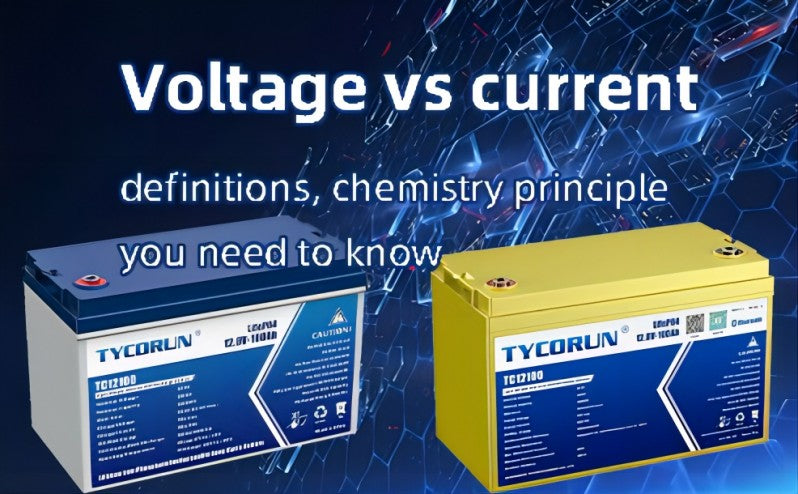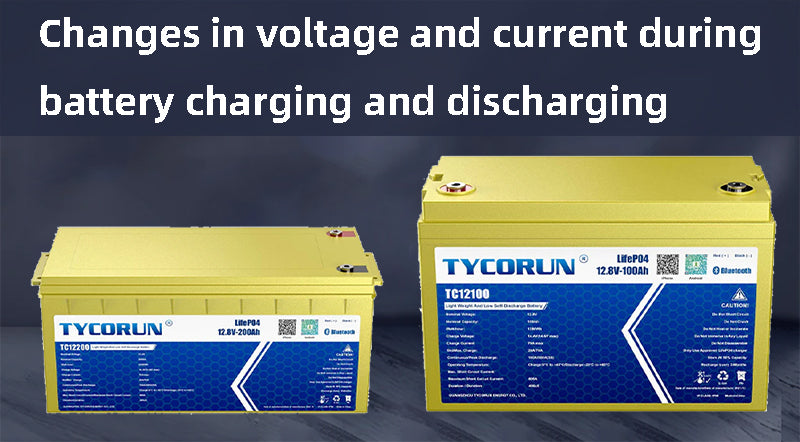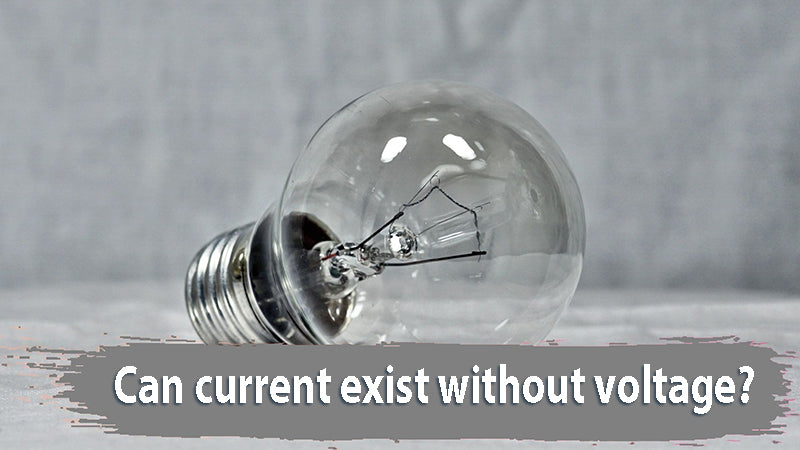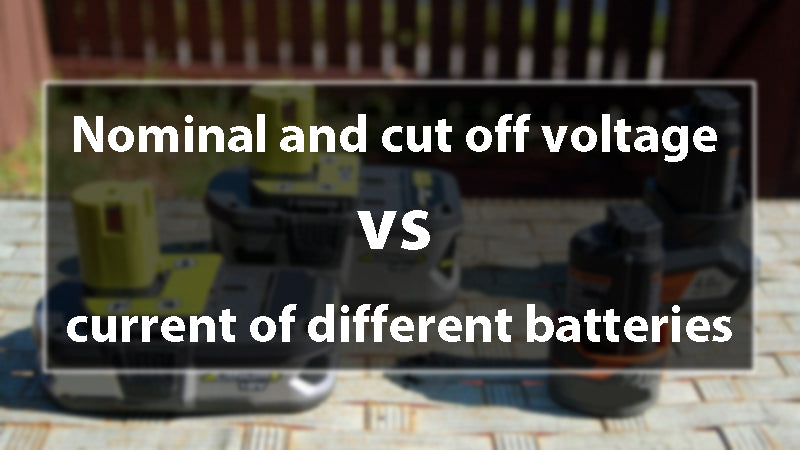Where there is voltage, there is current. It is because voltage is basically a push that causes charged particles to flow in a specific direction leading towards current generation. This current then drives a circuit with electricity. Undoubtedly, voltage and current are interrelated but these are two different terms to address. Voltage vs current is a comprehensive topic of physics. This article aims to figure it out and give answers.

## What is voltage and current?

 Voltage Current Voltage is also known as the electromotive force or emf. It is actually defined as the potential difference across two differently charged points in an electric field. Energy per unit charge can also be its definition. Current is defined as the rate at which the charged particles flow along the electric circuit. It is basically the result of the pressure generated at the positive & negative battery terminal, i.e., the electromotive force (force that cause the electrons to move)

Voltage vs current – the differences: Although voltage and current coexist at a place, they still have some differences. The discussing about voltage vs current elaborates on their differences and further verifies the above-mentioned statement. Below is a complete discussion related to “voltage vs current” which will help to clarify all the confusion concerning it.

 Current Voltage Formula I= V/R V= IR Relation Without voltage, current doesn’t flow Voltage can still be there in case of no current Unit Ampere Volt Measuring tool Ammeter Voltmeter Field generation Magnetic field Electric field Behavior in series connection Same in all battery cells of series circuits Distributed across components of series circuit Behavior in parallel connection Distributed over components in parallel circuit Same in battery cells parallel circuit## Changes in voltage and current during battery charging and discharging

There are basically two types of voltage, i.e., open circuit voltage OCV & closed-circuit voltage CCV. Open circuit voltage develops as a result of the series of chemical reactions occurring in electrolytes or metals. OCV changes with the type of metal and electrolyte used. Charging and discharging of the battery shifts the circuit from OCV to CCV. Charging raises the voltage, and discharging lowers the voltage. Now let's see how voltage vs current

• Discharging: The battery voltage gradually decreases, so the current gradually decreases. The discharge cut-off voltage of lithium iron phosphate battery is 2v.
• Charging: The battery voltage is gradually increased, and the charging current is gradually decreased. When the voltage is equal to the supply voltage, the idealized current is 0, and there will actually be very little leakage current. The discharge current refers to the normal load. If it is a constant current load, within a certain voltage range, the current does not change with the voltage. Similarly, if charging with constant current, the current is constant within a certain voltage range. This is one of the difference between voltage vs current.

## What is the chemistry principle that lies at the back of voltage and current?

The chemical principle behind the current is that the substance loses electrons in the reaction, and the other part gains electrons. During the process of transferring these electrons in the medium, an electric current is formed. The chemical principle of voltage is that substances lose electrons, so some substances gain electrons.

The potential difference between these two substances is called voltage. And there is another relationship of voltage vs current. Ohm’s law is the basic principle that discusses over voltage and current simultaneously. It states that current flowing through a circuit is directly related to the voltage or potential difference applied across the terminals of the battery. There is a formula: V= IR (where V= voltage, I= Current and R= Resistance)

## How voltage and current are measured?

Measuring the voltage and current requires a very simple process. Measuring instruments are used to measure voltage and current across a circuit. Both use two different measuring tools. Current is measured by ammeter and voltage by voltmeter. However, these two also come together in a single device known as “Multimeter” which can give readings after measuring voltage and current.

To measure voltage, apply multimeter in parallel series to the circuit. For current, it is attached in series. If it is a lithium battery with a smart BMS installed, you can directly see the battery status information such as voltage and current soc from the BMS.

Voltage is the reason for the formation of current, when the voltage difference is 0, no current will flow through the conductor, that is, the current is also 0. When the resistance is constant, the higher the voltage applied across the conductor, the greater the current through the conductor. When the voltage across both ends is constant, the greater the resistance of the conductor, the smaller the current flowing through it. Both voltage and current together determine current, so current is related to voltage. So when we compare voltage vs current, we can say that they have close relationship.## Can current exist without voltage? If not, then why?

No, current can not exist without voltage. It is because the current is basically the flow of charged particles which needs a force known as emf to push them forward. Voltage is actually that force. Without this force, current can’t flow. So, one can find voltage in a circuit with no current flow but can’t find current flow with no voltage.

Yes, voltage is what makes current, and without voltage there is no current. If there is current, it means that it is a channel, and there must be voltage. If there is a voltage but the circuit is broken, there will be no current, but the voltage will not disappear, it will still exist.

## FAQs revolving around voltage vs current

#### Why current is low when voltage is high?

Current is put low in electricity lines to increase efficiency at high voltage. In other words, to compensate power losses through resistance, current is kept low at high voltage.

#### Does higher voltage mean more current? Does more current mean higher voltage?

According to ohms law, the claim in the question is true. It is because ohms law states that voltage is directly proportional to current keeping the resistance constant. So, it is true that high voltage means more current or more current means high voltage. However, another situation is that the higher the voltage, the lower the current.

This situation occurs under the premise of a certain power, the current vs voltage are inversely proportional, because the power P = IU. A typical example of this is the transformer. For example, in order to reduce line loss, people will increase the voltage emitted by the generator several times to reduce the current and reduce the loss of the transmission line.

#### Does voltage and current flow in the same direction?

Direction of current is mostly measured through right hand rule. But in case of a voltage source it is mostly the conventional or positive current which flows away from the positive terminal of the battery. In a DC circuit, current and voltage go in the same direction. In some AC circuits, when the load is a motor or a transformer, the directions of current and voltage are different. Thus, voltage vs current is quite different, and they even have different flowing direction.

#### Why is current 0 when voltage is 0?

In the event that the voltage is 0, current will cease to flow. Because of this, 0 amperes of current correspond to 0 volts.

#### Which one is more powerful, voltage vs current?

Neither voltage nor current is particularly powerful on its own, but when combined, the two can propel a circuit forward. This is the same point in voltage vs current.

## Do different batteries have the same voltage and current?

No, different types of batteries have different current and voltages. It totally depends on their internal resistance and detailed specifications.Nominal and cut off voltage of different batteries

 Nominal voltage Charge cut off voltage Lead acid battery 2.0V 2.7-2.8V Lithium battery 3.2-3.7V 4.2V Ni-Cr battery 1.2V 1.45V NIMH battery 1.2V 1.25V

Different application scenarios require different volts of battery. Volts will change when in different devices. Do you know how mucn volts is a car battery or golf cart bttery? Go read our article!

## How does the voltage and current change in the case of series and parallel?

When the cells are connected in series, the capacity of the battery pack remains unchanged and the voltage increases; when the cells are connected in parallel, the voltage of the battery pack remains unchanged and the battery capacity increases.

## How do voltage and current affect the performance of battery?

Batteries with a higher voltage and the same mAh rating will have a longer lifespan due to the increased power.

## How to design an electrical system according to voltage and current?

An electrical system can be configured in either parallel or series, and these are the two best possible combinations for designing an electrical system. Before designing a circuit system based on voltage and current, we should calculate our power requirements. If you want high power, you need a larger voltage to support it. The higher the voltage, the smaller the current, which can better ensure the safety of the power system. Make sure the voltage is within a appropriate range with the current circuit voltage.

Related article: voltage in an aaa battery,